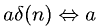Equations > Signal Processing > Fourier Transform Pairs > Discrete-Time Fourier transform of delta

### Discrete-Time Fourier transform of deltaLatex Code:

MathML Code:

 $a\delta \left(n\right)⇔a$

MathType 5.0: